# C++ this指针和空指针

### this指针和空指针

C++通过提供特殊的对象指针,this指针，解决上述问题。this指针指向被调用的成员函数所属的对象

this指针是隐含每一个非静态成员函数内的—种指针
this指针不需要定义，直接使用即可

this指针的用途:
*·当形参和成员变量同名时，可用this指针来区分
·在类的非静态成员函数中返回对象本身，可使用return this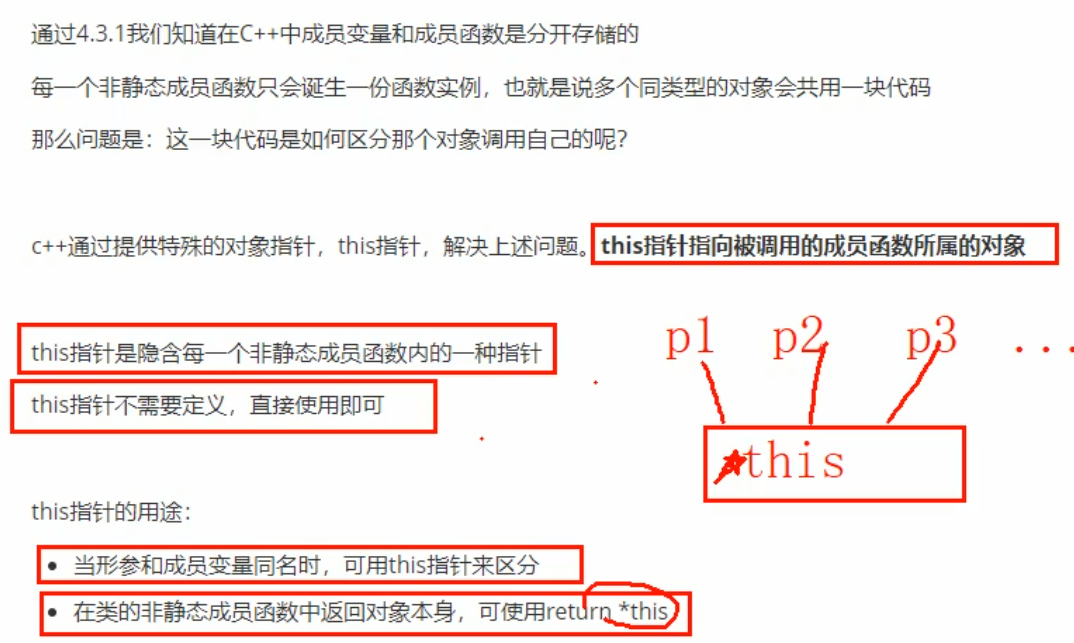# 一、this指针

## 1 this指针解决名称冲突

``````#include <iostream>
using namespace std;
class Person
{
public:
Person(int age)
{
age = age;
}
int age;

};
//1 解决名称冲突
void test1()
{
Person p1(18);
cout << "p1的年龄为=" << p1.age << endl;
}
int main()
{
test1();
return 0;
}
``````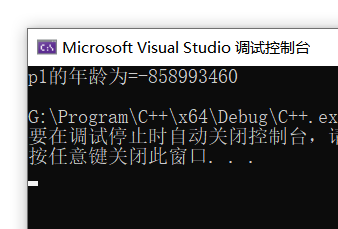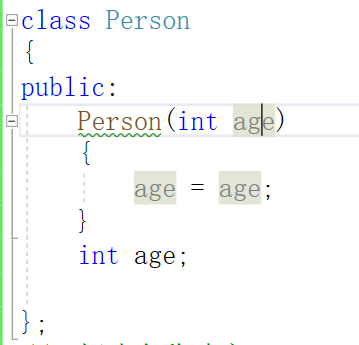``````class Person
{
public:
Person(int age)
{
m_Age = age;
}
int m_Age;

};
``````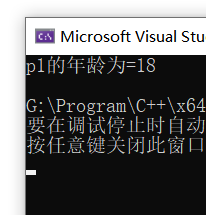this指针指向 被调用的成员函数 所属对象

``````class Person
{
public:
//1 解决名称冲突
Person(int age)
{
//this指针指向 被调用的成员函数 所属对象
this->age = age;
}
int age;

};
``````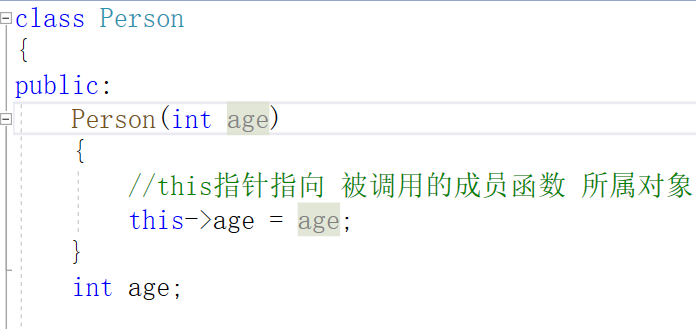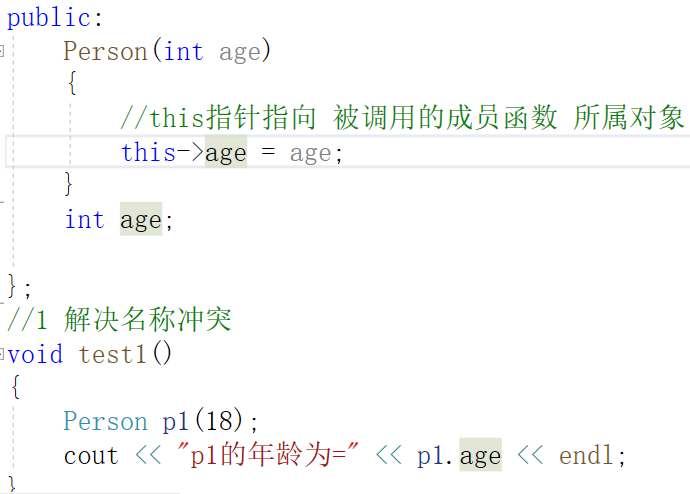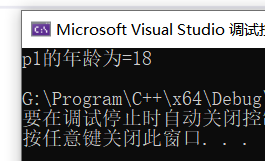## 2 返回对象本身用*this

``````class Person
{
public:
//1 解决名称冲突
Person(int age)
{
//this指针指向 被调用的成员函数 所属对象
this->age = age;
}

//2 返回对象本身用*this
{
this->age += p.age;
}

int age;

};
//1 解决名称冲突
void test1()
{
Person p1(18);
cout << "p1的年龄为=" << p1.age << endl;

//2 返回对象本身用*this
Person p2(10);
cout << "p2的年龄为=" << p2.age << endl;
}
``````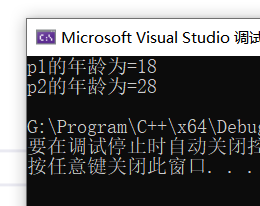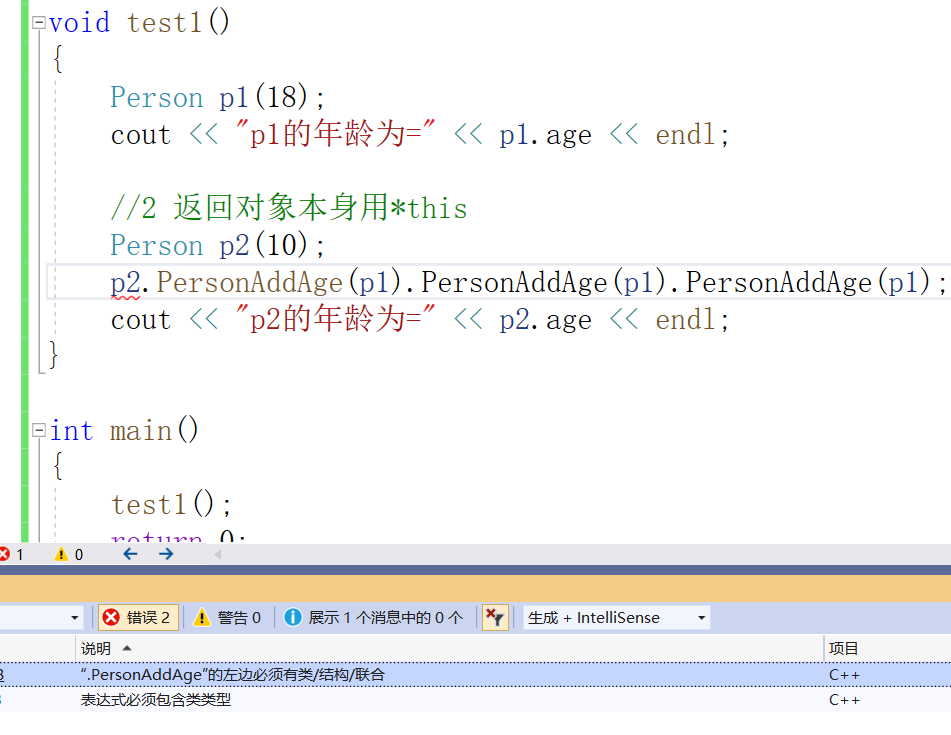``````//2 返回对象本身用*this
{
//this指向p2的指针，而*this指向的就是p2这个对象的本体
this->age += p.age;
return *this;
}
``````

``````#include <iostream>
#include<string>
using namespace std;

//1 解决名称冲突

//2 返回对象本身用*this

class Person
{
public:
//1 解决名称冲突
Person(int age)
{
//this指针指向 被调用的成员函数 所属对象
this->age = age;
}

//2 返回对象本身用*this
{
//this指向p2的指针，而*this指向的就是p2这个对象的本体
this->age += p.age;
return *this;
}

int age;

};
//1 解决名称冲突
void test1()
{
Person p1(18);
cout << "p1的年龄为=" << p1.age << endl;

//2 返回对象本身用*this
Person p2(10);
cout << "p2的年龄为=" << p2.age << endl;
}

int main()
{
test1();
return 0;
}
``````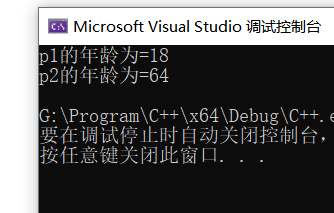# 二、空指针调用成员函数

C++中空指针也是可以调用成员函数的，但是也要注意有没有用到this指针

``````#include<iostream>
using namespace std;

//空指针调用成员函数

class Person
{
public:
void showClassName()
{
cout << "This is Person class" << endl;
}

void showPersonAge()
{
//报错原因是因为传入的是空指针
cout << "age=" <<m_Age<< endl;
}
int m_Age;
};

void test1()
{
Person* p = NULL;
p->showClassName();
//	p->showPersonAge();//报错原因是因为传入的是空指针
}

int main()
{
test1();
return 0;
}
``````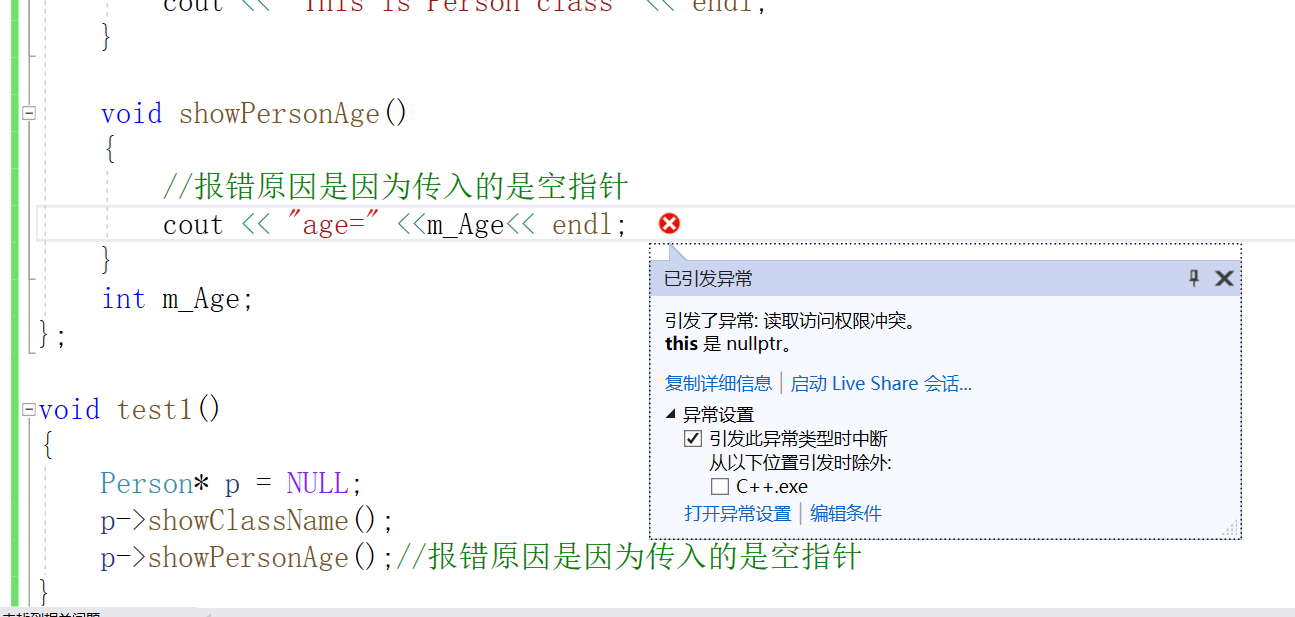//报错原因是因为传入的是空指针

if(this==NULL) return; //解决空指针出错

``````void showPersonAge()
{
//报错原因是因为传入的是空指针

if(this==NULL)  return; //解决空指针出错

cout << "age=" <<m_Age<< endl;
}
``````

``````#include<iostream>
using namespace std;

//空指针调用成员函数

class Person
{
public:
void showClassName()
{
cout << "This is Person class" << endl;
}

void showPersonAge()
{
//报错原因是因为传入的是空指针

if(this==NULL)  return; //解决空指针出错

cout << "age=" <<m_Age<< endl;
}
int m_Age;
};

void test1()
{
Person* p = NULL;
p->showClassName();
p->showPersonAge();//报错原因是因为传入的是空指针
}

int main()
{
test1();
return 0;
}
``````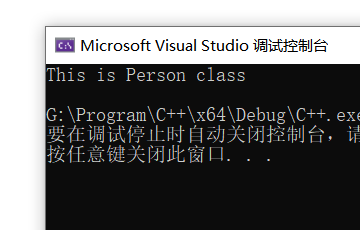# 三、const修饰成员函数

·成员函数后加const后我们称为这个函数为常函数
.常函数内不可以修改成员属性
·成员属性声明时加关键字mutable后，在常函数中依然可以修改

·声明对象前加const称该对象为常对象
·常对象只能调用常函数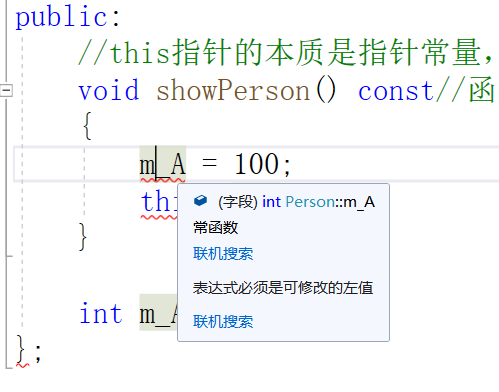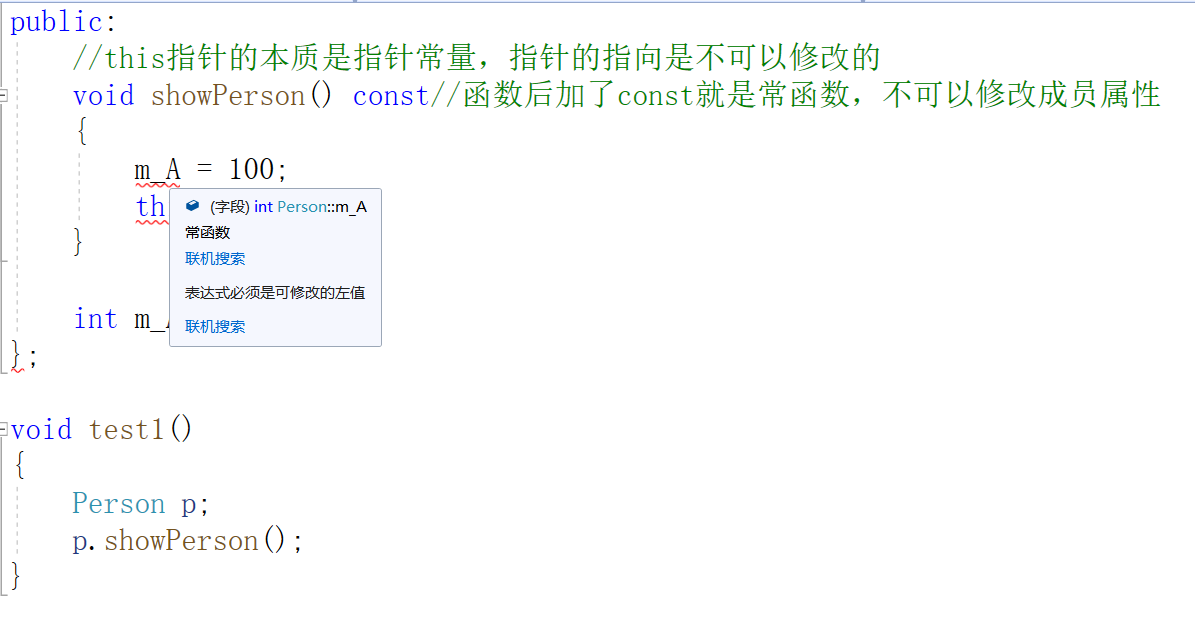mutable int m_B;//特殊变量，即使在常函数中，也可修饰这个值，加关键字mutable

``````//常函数
class Person
{
public:
//this指针的本质是指针常量，指针的指向是不可以修改的
//const Person *const this;
//在成员函数后面加const，修饰的是this指向，让指针指向的值也不可以修改
void showPerson() const
{
//	m_A = 100; //常函数内不可以修改成员属性
//	this->m_A = 100;
//	this = NULL;
m_B = 100; //成员属性声明时加关键字mutable，在常函数中才可以修改
}

int m_A;
mutable int m_B;//特殊变量，即使在常函数中，也可修饰这个值，加关键字mutable
};
``````

const Person p;//在对象前加const变常对象

``````//常对象
void test2()
{
const Person p;//在对象前加const变常对象
//	p.m_A = 100;//报错
p.m_B = 100;//m_B是特殊值，在常对象下也可以修改

//常对象只能调用常函数
p.showPerson();
//	p.func();//常对象不可以调用普通成员函数，因为普通成员函数可以修改属性
}
``````

``````#include<iostream>
using namespace std;

//常函数
//常对象

class Person
{
public:
//this指针的本质是指针常量，指针的指向是不可以修改的
//const Person *const this;
//在成员函数后面加const，修饰的是this指向，让指针指向的值也不可以修改
void showPerson() const
{
//	m_A = 100; //常函数内不可以修改成员属性
//	this->m_A = 100;
//	this = NULL;
m_B = 100; //成员属性声明时加关键字mutable，在常函数中才可以修改
}

void func()
{

}

int m_A;
mutable int m_B;//特殊变量，即使在常函数中，也可修饰这个值，加关键字mutable
};
//常函数
void test1()
{
Person p;
p.showPerson();
}

//常对象
void test2()
{
const Person p;//在对象前加const变常对象
//	p.m_A = 100;//报错
p.m_B = 100;//m_B是特殊值，在常对象下也可以修改

//常对象只能调用常函数
p.showPerson();
//	p.func();//常对象不可以调用普通成员函数，因为普通成员函数可以修改属性
}
int main()
{
test1();
return 0;
}
``````

；原文链接：https://blog.csdn.net/m0_51233386/article/details/116163585

• 周排行
• 月排行
• 总排行

## 随机推荐

• ### MVC4制作网站教程第三章 修改用户组操作3

一、用户 二、用户组 2.1浏览用户组 2.2添加用户组 2.3修改用户组 首先打开【Use...

• ### 奇妙的文件系统对象组件

翻译 讨饭猫 很久以前（其实就几个月）就知道 Chinaasp 有个实际效果象黑客的ASE...

• ### ASP中获得Select Count语句返回值的方法

我们一般统计数据库记录时会用到Select Count(*)语句，当我们使用SQL Server的查...

• ### 测试你对技术的掌握度：JSP程序员成长之

文/bingo_ge 在本文章中使用精通、熟练、熟悉、了解标志你对某技术的掌握程度。 ...

• ### 6年Java老鸟聊聊新人到底要不要学Java，

6年Java老鸟聊聊新人到底要不要学Java从事互联网《打工人的那些事》 文章目录 为...

• ### HTML制作个人简历的简单实现

简历代码： XML/HTML Code 复制内容到剪贴板 !DOCTYPEhtmlPUBLIC-//W3C//DTDXHTM...

• ### js数组的基本使用总结

前言 数组是一种特殊对象。js中并没有真正的数组，只是用对象模拟数组。 查看对...

• ### Windows Terminal完整指南

在本文中，我们将探讨Windows Terminal，它是WSL2的理想配套。它速度快、可配置...

• ### 微信小程序实现简单的select下拉框

微信小程序实现一个简单的select下拉框，供大家参考，具体内容如下 用的是transf...

• ### 详解VSCode打开多个项目文件夹的解决方法

最近从sublime转vscode，自然而然就会把sublime的一些习惯带过来，其中有一点让...